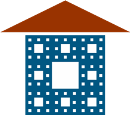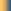Department of Computer Science & Mathematics

Topology Research Group

Nipissing UniversityMay 14-18, 2012 Workshop on Recent Advances in General Topology, Dimension Theory, Continuum Theory and Dynamical Systems Schedule and Abstracts Workshop homepage

Welcome Party - May 13 at 6 PM
Pizza and beer in the Governor’s house assembly room.

Dinner - May 16 at 6:30 PM
Buffet dinner with cash bar in the small cafeteria (A246)

Schedule
Room: A226

 Monday May 14 Tuesday May 15 Wednesday May 16 Thursday  May 17 Friday May 18 9:30 - 10:30 Van Mill Van Mill Minc Minc Minc 10:30  11:00 break break break break break 11:00  12:00 Antonyan Kibedi Martinez Oversteegen Mayer 12:00  13:30 lunch lunch lunch lunch lunch 13:30  14:30 Tymchatyn Steprans Hoehn Ptacek TBA 15:00  16:00 Q&A session Q&A session Q&A session Q&A session Q&A session

Abstracts

Sergey Antonyan (UNAM, Mexico)

The Gromov-Hausdorff Hyperspaces

Click for abstract

Logan Hoehn (U. of Alabama- Birmingham)

Shortest paths in plane domains

I will consider problems concerning shortest paths joining two points in the boundary of a plane domain.  In particular, I will outline the construction of a shortest path in any given homotopy class relative to the endpoints.  Along the way, I will introduce an alternative notion of path length to facilitate the study of shortest paths in the absence of any path of finite Euclidean length joining the two points in question.

Francisco Kibedi (York University)

Saturated linear orders and Martin's axiom

Click for abstract

Veronica Martinez (UNAM)

Jan van Mill (VU-Amsterdam)

Various aspects of countable dense homogeneity

We prove that a connected, countable dense homogeneous space is n-homogeneous for every n, and strongly 2-homogeneous provided it is locally connected. We also present an example of a connected and countable dense homogeneous space which is not strongly 2-homogeneous. We prove that a countable dense homogeneous space has size at most continuum. If it moreover is compact, then it is first-countable under the Continuum Hypothesis. We also construct under the Continuum Hypothesis an example of a hereditarily separable, hereditarily Lindelof, countable dense homogeneous compact space of uncountable weight. Some of the presented results were obtained with A.V. Arhangelskii.

Piotr Minc (Auburn University)

Combinatorics and topology of simplicial maps of graphs

A simplicial graph $G$ is a (geometric) graph with if its set of vertices fixed. Two vertices of $G$ are adjacent if they belong to the same edge. For $G$ and $H$ simplicial graphs, a function from the set of vertices of $H$ into the set of vertices of $G$ is simplicial if any two adjacent vertices are taken either to one vertex or to a pair of adjacent vertices. Each simplicial function can be linearly A simplicial graph is an arc (a tree) if its geometric representation is a topological arc (a topological tree). We say that a simplicial map $\varphi:G\to H$ can be factored through an arc if there is a simplicial arc $A$ and there are two simplicial maps $\alpha:G\to A$ and $\beta:A\to H$ such that $\varphi=\beta\circ\alpha$. We will talk about combinatorics of factorization through an arc and its role in many continuum theory problems including embedding into the plane, fixed point problems, and nonchainability of inverse limits of trees with simplicial bonding maps.

Lex Oversteegen (U. of Alabama-Birmingham)

The structure of the space of invariant laminations

Joint work with: A.Blokh, R. Ptacek and V. Timorin.

Laminations were introduced by Thurston. Laminations  are closed collections of chords, called leaves, of the unit disk in the complex plane  which meet at most in endpoints (we do allow for degenerate leaves; i.e., points in the unit circle). We denote a leaf by its endpoints in the unit circle as $\overline{ab}$ A lamination is  $d$-invariant  (under the covering map $\sigma_d(z)=z^d$ on the unit circle) if for every leaf $\overline{ab}$ the chord $\overline{\sigma_d(a)\sigma_d(b)}$ is also a leaf,  for every leaf $\ell$ with non-degenerate image $\ell'$ there exist exactly $d$ pairwise disjoint leaves $\{\ell=\ell_1,\dots,\ell_d\}$ all of which map onto the leaf $\ell'$ and every leaf has at least one pre-image leaf. Thurston showed that a quotient of the space of $2$-invariant laminations is itself a lamination. In this talk we will focus on the spaces of $2$- and $3$-invariant laminations.

Ross Ptacek (U. of Alabama-Birmingham )

Laminations from the Cubioids

Click for abstract

Juris Steprans (York University)

Amenable group actions

It is known that if the action of a compact group  on itself has an invariant mean, this mean is unique. The same is not true, however, for the action of a group on a set. This talk will survey results in this area, mention some new results and pose questions whose further study may prove fruitful.

Nonseparating subcontinua of planar curves

Joint work with Dale Daniel.

It is a classical theorem that every non-degenerate continuum contains at least two points neither of which separates the continuum. It was a question of Y.T. Leung whether each planar continuum contained a non-degenerate subcontinuum which does not separate the continuum. Nadler and Seldomridge constructed a dendroid (i.e. hereditarily unicoherent, arcwise connected subcontinuum) X in R^3 in which every non-degenerate subcontinuum separates X. We prove that every continuum in which Leung's question has a negative answer is suslinian (i.e. contains no uncountable collection of pairwise disjoint, non-degenerate subcontinua). We also prove that  each hereditarily unicoherent planar continuum  Y contains a non-degenerate proper subcontinuum which fails to separate Y.Group HomeGroup MembersPopular LecturesNUMERICFields InstituteNSERCTopology AtlasArxiv.orgThe Geometry Center